# Extension of a field

(diff) ← Older revision | Latest revision (diff) | Newer revision → (diff)
Jump to: navigation, search

field extension

A field containing the given field as a subfield. The notation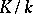means thatis an extension of the field. In this case,is sometimes called an overfield of the field.

Letand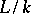be two extensions of a field. An isomorphism of fields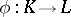is called an isomorphism of extensions (or a-isomorphism of fields) ifis the identity on. If an isomorphism of extensions exists, then the extensions are said to be isomorphic. If,is called an automorphism of the extension. The set of all automorphisms of an extension forms a group,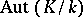. Ifis a Galois extension, this group is denoted byand is called the Galois group of the fieldover, or the Galois group of the extension. An extension is called Abelian if its Galois group is Abelian.

An elementof the fieldis called algebraic overif it satisfies some algebraic equation with coefficients in, and transcendental otherwise. For every algebraic elementthere is a unique polynomial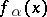, with leading coefficient equal to 1, that is irreducible in the polynomial ring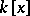and satisfies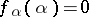; any polynomial overhavingas a root is divisible by. This polynomial is called the minimal polynomial of. An extensionis called algebraic if every element ofis algebraic over. An extension that is not algebraic is called transcendental. An extension is called normal if it is algebraic and if every irreducible polynomial inhaving a root infactorizes into linear factors in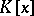. The subfieldis said to be algebraically closed inif every element ofthat is algebraic overactually lies in, that is, every element of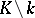is transcendental over. A field that is algebraically closed in all its extensions is called an algebraically closed field.

An extensionis said to be finitely generated (or an extension of finite type) if there is a finite subsetofsuch thatcoincides with the smallest subfield containingand. In this case one says thatis generated byover. Ifis generated overby one element, then the extension is called simple or primitive and one writes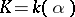. A simple algebraic extension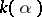is completely determined by the minimal polynomial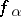of. More precisely, if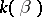is another simple algebraic extension and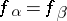, then there is an isomorphism of extensions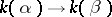sendingto. Furthermore, for any irreducible polynomial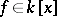there is a simple algebraic extensionwith minimal polynomial. It can be constructed as the quotient ring. On the other hand, for any simple transcendental extensionthere is an isomorphism of extensions, whereis the field of rational functions inover. Any extension of finite type can be obtained by performing a finite sequence of simple extensions.

An extensionis called finite ifis finite-dimensional as a vector space over, and infinite otherwise. The dimension of this vector space is called the degree ofand is denoted by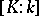. Every finite extension is algebraic and every algebraic extension of finite type is finite. The degree of a simple algebraic extension coincides with the degree of the corresponding minimal polynomial. On the other hand, a simple transcendental extension is infinite.

Suppose one is given a sequence of extensions. Then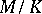is algebraic if and only if bothand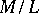are. Further,is finite if and only ifandare, and thenIf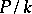andare two algebraic extensions and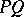is the compositum of the fieldsandin a common overfield, then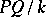is also algebraic.

How to Cite This Entry:
Extension of a field. Encyclopedia of Mathematics. URL: http://encyclopediaofmath.org/index.php?title=Extension_of_a_field&oldid=13369
This article was adapted from an original article by O.A. Ivanova (originator), which appeared in Encyclopedia of Mathematics - ISBN 1402006098. See original article